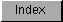```
The IEEE double-precision variables are defined as 64-bit entities with
the following format:

______________________________________________
|                                              |
| SEEEEEEE    EEEEEIMM    MMMMMMMM    MMMMMMMM |
| 63          55          47          39       |
|______________________________________________|

______________________________________________
|                                              |
| MMMMMMMM    MMMMMMMM    MMMMMMMM    MMMMMMMM |
| 31          23          15          7        |
|______________________________________________|

Hidden Bit In The Mantissa.
---------------------------
There is a "hidden" bit in the mantissa part of the IEEE numbers.
Since all numbers are normalized, the integer (high) bit of the
mantissa is dropped off.  The IEEE double-precision range is 2.2E-308
(4.9E-324 de-normalized) to 1.8E+307.

The exponent is the power of two needed to correctly position the mantissa
to reflect the number's true arithmetic value.  If both the exponent and
the mantissa have zero in every position, the value is zero.  If only the
exponent has zero in every position, the value is an unnormal (extremely
small).  If all bits of the exponent are set to 1 the value is either a
positive or negative infinity or a Not a Number (NaN).  NaN is sometimes
used to indicate an uninitialized variable.

```